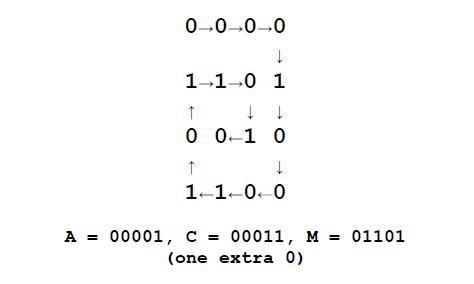## Encoding

#### 문제

Chip and Dale have devised an encryption method to hide their (written) text messages. They first agree secretly on two numbers that will be used as the number of rows (R$R$) and columns (C$C$) in a matrix. The sender encodes an intermediate format using the following rules:

1. The text is formed with uppercase letters [A-Z] and space.
2. Each text character will be represented by decimal values as follows: space = 0, A = 1, B = 2, C = 3, ..., Y = 25, Z = 26

The sender enters the 5 digit binary representation of the characters’ values in a spiral pattern along the matrix as shown below. The matrix is padded out with zeroes (0) to fill the matrix completely. For example, if the text to encode is: "ACM" and R$R$=4 and C$C$=4, the matrix would be filled in as follows:The bits in the matrix are then concatenated together in row major order and sent to the receiver. The example above would be encoded as: 0000110100101100

#### 입력

The first line of input contains a single integer N\,(1 \le N \le 1000)$N\,(1 \le N \le 1000)$ which is the number of datasets that follow.

Each dataset consists of a single line of input containing R\,(1 \le R\le 21)$R\,(1 \le R\le 21)$, a space, C\,(1 \le C \le 21)$C\,(1 \le C \le 21)$,
a space, and a text string consisting of uppercase letters [A-Z] and space. The length of the text string is guaranteed to be \le (R\times C)/5$\le (R\times C)/5$.

#### 출력

For each dataset, you should generate one line of output with the following values: The dataset number as a decimal integer (start counting at one), a space, and a string of binary digits (R \times C)$(R \times C)$ long describing the encoded text. The binary string represents the values used to fill in the matrix in row-major order. You may have to fill out the matrix with zeroes (0) to complete the matrix.

#### 예제 입력

4
4 4 ACM
5 2 HI
2 6 HI
5 5 HI HO

#### 예제 출력

1 0000110100101100
2 0110000010
3 010000001001
4 0100001000011010110000010